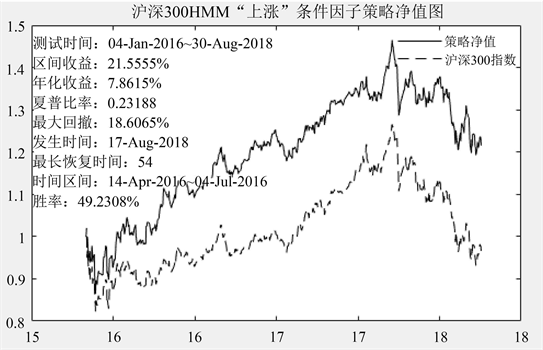﻿ 基于条件随机场的量化选股模型 Quantitative Stock Picking Model Based on Conditional Random Fields

Statistics and Application
Vol. 08  No. 02 ( 2019 ), Article ID: 29721 , 7 pages
10.12677/SA.2019.82034

Quantitative Stock Picking Model Based on Conditional Random Fields

Yusi Zhang, Manfa Liang

School of Mathematics, South China University of Technology, Guangzhou GuangdongReceived: Mar. 25th, 2019; accepted: Apr. 9th, 2019; published: Apr. 16th, 2019ABSTRACT

As a special Conditional Random Field model, the Hidden Markov Model is one of the secret weapons behind the brilliant performance of the medallion fund. From the perspective of financial engineering, this paper introduces the Hidden Markov Model into the investment field to predict the future rising and falling situation of individual stocks. Assuming that each of the ups and downs has a clear pattern, which can be described by an HMM model, if the probability of a stock's observation on the HMM model characterizing the rising pattern is greater, the probability that the stock actually rises is also the bigger. Based on the HMM model, the HMM factor of individual stocks was constructed, and the empirical analysis of the historical back testing of the Shanghai and Shenzhen 300 constituent stocks from 2016 to 2018 achieved a good excess return, which indicated that the conditional random field introduced into the quantitative stock selection has a predictive ability.

Keywords:Conditional Random Field, Financial Engineering, HMM, Rising Pattern1. 引言

2. 隐马尔可夫模型

2.1. 基本假设

${b}_{j}\left({o}_{t}\right)=P\left({o}_{t}|{X}_{t}=j\right)$ (1)

1) $S=\left\{1,\cdots ,N\right\}$ 为状态集合，在时刻 $t$ 的状态定义为 ${s}_{t}$

2) $K=\left\{{k}_{1},\cdots ,{k}_{M}\right\}$ 为输出字母，在离散的观测密度下， $M$ 是观测可选择的数目，

3) 初始状态分布 $\Pi =\left\{{\text{π}}_{i}\right\},i\in S$${\text{π}}_{i}$ 定义为

${\text{π}}_{i}=P\left({s}_{1}=i\right)$ (2)

4) 状态转移概率分布 $A=\left\{{a}_{ij}\right\},\text{\hspace{0.17em}}i,j\in S$

${a}_{ij}=P\left({s}_{t+1}=j|{s}_{t}=i\right),1\le i,j\le N$ (3)

5) 观测符号概率分布 $B={b}_{j}\left({o}_{t}\right),j\in S$ 。对于每一个状态 $j$ 产生观测的概率函数为：

${b}_{j}\left({o}_{t}\right)=P\left({o}_{t}|{s}_{t}=j\right)$ (4)

2.2. 前向算法

${\alpha }_{i}\left(t\right)=P\left({o}_{1}{o}_{2}\cdots {o}_{t-1},{s}_{t}=i|\mu \right)$ (5)

${\alpha }_{i}\left(t\right)$ 保存在给定观测序列 ${o}_{1}\cdots {o}_{t-1}$ 时刻 $t$ 状态为 $i$ 的总概率。它是通过对网格节点处所有传入弧的概率求和来计算的。在每一个时刻 $t$ 的前向变量能够通过归纳法计算，流程如下：

1) 初始化：

${\alpha }_{i}\left(1\right)={\text{π}}_{i},1\le i\le N$ (6)

2) 归纳：

${\alpha }_{j}\left(t+1\right)=\underset{i=1}{\overset{N}{\sum }}{\alpha }_{i}\left(t\right){a}_{ij}{b}_{j}\left({o}_{t}\right),1\le t\le T,1\le j\le N$ (7)

3) 更新时间 $t=t+1$ ；如果 $t 返回第2步；否则终止算法。

4) 计算：

$P\left(O|\mu \right)=\underset{i=1}{\overset{N}{\sum }}{\alpha }_{i}\left(T\right)$ (8)

2.3. Viterbi算法

Viterbi算法被研究用来寻找在给定观测序列 $O=\left({o}_{1},{o}_{2},\cdots ,{o}_{T}\right)$ 最可能的状态序列 ${S}^{\prime }=\left({s}_{1},{s}_{2},\cdots ,{s}_{T}\right)$ $\underset{{S}^{\prime }}{\mathrm{arg}\mathrm{max}}P\left({S}^{\prime }|O,\mu \right)$ ；对于固定的观测序列最大化 $\underset{{S}^{\prime }}{\mathrm{arg}\mathrm{max}}P\left({S}^{\prime },O|\mu \right)$ 就足够了。

${\delta }_{j}\left(t\right)=\underset{{s}_{1}\cdots {s}_{t-1}}{\mathrm{max}}P\left({s}_{1}\cdots {s}_{t-1},{o}_{1}\cdots {o}_{t},{s}_{t}=j|\mu \right)$ (9)

1) 初始化

${\delta }_{i}\left(1\right)={\text{π}}_{i}{b}_{i}\left({o}_{1}\right),1\le i\le N$ (10)

${\psi }_{i}\left(1\right)=0,1\le i\le N$ (11)

2) 归纳

${\delta }_{j}\left(t\right)={b}_{j}\left({o}_{t}\right)\underset{1\le i\le N}{\mathrm{max}}{\delta }_{i}\left(t-1\right){a}_{ij}$ (12)

${\psi }_{j}\left(t\right)=\underset{1\le i\le N}{\mathrm{arg}\mathrm{max}}\left[{\delta }_{i}\left(t-1\right){a}_{ij}\right]$ (13)

3) 更新时间

$t=t+1$ (14)

4) 结束

${P}^{*}=\underset{1\le i\le N}{\mathrm{max}}\left[{\delta }_{i}\left(T\right)\right]$ (15)

${s}_{T}^{*}=\underset{1\le i\le N}{\mathrm{arg}\mathrm{max}}\left[{\delta }_{i}\left(T\right)\right]$ (16)

5) 路径回读

${s}_{t}^{*}={\psi }_{t+1}\left({s}_{t+1}^{*}\right)$ (17)

2.4. Baum-Welch算法

${p}_{t}\left(i,j\right)=P\left({s}_{t}=i,{s}_{t+1}=j|O,\mu \right)$ (18)

${\gamma }_{i}\left(t\right)=P\left({s}_{t}=i|O,\mu \right)={\sum }_{j=1}^{N}P\left({s}_{t}=i,{s}_{t+1}=j|O,\mu \right)={\sum }_{j=1}^{N}{p}_{t}\left(i,j\right)$ (19)

1) 初始化：随机选择 ${a}_{ij}^{\left(0\right)}$${b}_{j}^{\left(0\right)}\left(k\right)$${\text{π}}_{i}^{\left(0\right)}$ ，得到模型 ${\mu }^{\left(0\right)}=\left({A}^{\left(0\right)},{B}^{\left(0\right)},{\text{π}}^{\left(0\right)}\right)$

2) 归纳

${a}_{ij}^{\left(n+1\right)}=\left({\sum }_{t=1}^{T}{p}_{t}\left(i,j\right)\right)/\left({\sum }_{t=1}^{T}{\gamma }_{i}\left(t\right)\right)$ (20)

${b}_{i}^{\left(n+1\right)}\left(k\right)=\left({\sum }_{t:{o}_{t}=k,1\le t\le T}{\gamma }_{i}\left(t\right)\right)/\left({\sum }_{t=1}^{T}{\gamma }_{i}\left(t\right)\right)$ (21)

${\text{π}}_{i}={\gamma }_{i}\left(1\right)$ (22)

3) 更新：

$n=n+1$ (23)

4) 结束：得出模型的最终参数为 ${\mu }^{\left(n+1\right)}=\left({A}^{\left(n+1\right)},{B}^{\left(n+1\right)},{\text{π}}^{\left(n+1\right)}\right)$

3. 实证分析

3.1. HMM训练过程

1) 选取数据：假设观测序列长度为 $k$ ，那么将每个股票的技术面信息以及基本面信息切分成日频的观测序列，每一日的信息向量作为特征向量对应HMM语音识别模型中的每一个观测值向量，取 $t-k+1$$t$ 日的特征向量，获得长度为 $k$ 的观测序列，对应HMM模型中的每一个观测序列。

2) 训练样本：对数据样本进行上涨与下跌模型样本的分割。在模型训练时，我们选择 $t+m$ 日股价相对 $t$ 日上涨的股票作为上涨模型的训练样本，根据多观测序列的训练算法训练出上涨模型的连续观测序列HMM参数；选择 $t+m$ 日股价相对于 $t$ 日下跌的股票作为下跌模型的训练样本，根据多观测序列的训练算法训练出下跌模型的连续观测序列HMM参数；

3) 模型预测：在进行实际预测时，在 $t$ 日，我们分别将每个股票在 $t-k+1$$t$ 日的信息向量即特征向量作为特征输入训练好的上涨模型和下跌模型中，计算出不同模型下观测到该观测序列的概率p(上涨)与 $p\left(下跌\right)$ 。如果p(上涨) > p(下跌)，则说明该观测序列由上涨模型生成的概率更大，该股票在 $t+m$ 日相对于 $t$ 日上涨的概率大于下跌的概率；反之，则认为该股票下跌的概率大于上涨的概率。

3.2. 策略设计及结果

1) 在调仓日 $t$ 时，计算出当日沪深300成分股全部个股的“上涨”条件因子；

2) 选股时去除ST、PT股，去除停牌股票，去除上市未满一年股票，去除每股收益小于0股票；

3) 采取行业中性按照行业进行选股，根据“上涨”条件因子数据从大到小排名对成分股进行选取，从而挑选出排名前20%的股票进行投资；

4) 根据成分股的比例计算出每一个行业的占比 ${m}_{i}$

5) 行业的股票等资金投入，第 $i$ 个行业有 $K$ 只股票投资，则行业的每只个股投入资金比例为 ${m}_{i}/K$

6) 根据资金情况以及个股收盘价计算出调仓日个股买入的仓位数量。

7) 计算日收益率、基准收益率；然后计算年化收益率、最大回撤、夏普比、最长修复时间等指标策略净值图如图1所示。Figure 1. Unhedged net worth chart of stock selection strategy based on HMM modelTable 1. The indicator of “Up” conditional factor selection strategy based on HMM model

4. 结论

HMM的思想引入到预测股票的趋势中，根据预测指标来构造一些有效的投资策略。根据股票价格趋势的动量性与持续性，股价走势在一段时间内，涨和跌的股票各自都存在一种明确的模式与其对应，都分别可由一个HMM模型来描述；同时股票的行情数据以及基本面数据表现例如换手率、收益率、股票市值可由有限个服从马尔可夫过程的隐状态所决定，且满足齐次马尔可夫假设和观测独立性假设。根据表1所示，运用HMM模型构造出的“上涨”条件因子进行选股策略中年化收益率为8.94%，最大回撤为8.61%，而指数年化收益为−1.33%，最大回撤为26.43%，策略的夏普比远远大于指数投资的夏普比，效果远远超过指数。用HMM模型进行选股更能促使策略抓住一致性趋势，给策略更好的抓准投资的时机，补充策略的缺陷与单一性。

Quantitative Stock Picking Model Based on Conditional Random Fields[J]. 统计学与应用, 2019, 08(02): 296-302. https://doi.org/10.12677/SA.2019.82034

1. 1. 郭平. 贝叶斯概率图像分割中混合模型参数高效计算的研究[J]. 计算机科学, 2002, 29(8): 101-103.

2. 2. 郭平, 卢汉清. 贝叶斯概率图像自动分割研究[J]. 光学学报, 2002, 22(12): 1479-1483.

3. 3. 郭松涛, 孙强, 等. 基于改进小波域隐马尔可夫模型的遥感图像分割[J]. 电子与信息报, 2005, 27(2): 286-289.

4. 4. 林亚忠. 基于Gibbs随机场模型的医学图像分割新算法研究[D]: [博士学位论文]. 广州: 第一军医大学, 2004.

5. 5. Zhang, L. and Ji, Q. (2010) Image Segmentation with a Unified Graphical Model. IEEE Transactions on Pattern Analysis and Machine In-telligence, 32, 1406-1425. https://doi.org/10.1109/TPAMI.2009.145

6. 6. Zhang, L. and Ji, Q. (2011) A Bayesian Network for Automatic and Interactive Image Segmentation. IEEE Transaction on Image Processing, 20, 2582-2593. https://doi.org/10.1109/TIP.2011.2121080